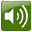<< Previous

Numbers 11 to 99

11
The number 11 is almost like the number one (waaHid) and ten (3ashara) except a tiny bit different. waaHid becomes 'aHid and 3ashara becomes 3ashar.
'aHidaa 3asharأَحِدى عَشَ - ١١

12
The number 12 is almost like the number two ('ithnaan) and ten (3ashara) except a tiny bit different. ithnaan becomes 'ithnaa.
'ithnaa 3ashar(a)إثنا عَشَ - ١٢

13 - 19
For the numbers 13-19 you can take the regular number (3-9) and add 3ashar.For example here are 13 to 15. Note how the taaʾ marbuuTah at the end of each number is pronounced as 't'.
 ثَلاثة عَشَ - ١٣أَربَعة عَشَ - ١٤خَمْسَة عَشَ - ١٥20
The number twenty is a little irregular but as you will see it is similar to other multiples of ten.
3ishriinعِشرين - ٢٠

30, 40, 50, 60 ...
All the numbers that are multiples of ten can be formed with these rules:
• Take a number from 3 to 9
• Remove the taaʾ marbuuTah
• Add 'iin' to the end (In some dialects it is 'uun' at the end)
For example here are 30, 40 and 50.
 ثَلاثين - ٣٠أَربَعين - ٤٠خَمْسِين - ٥٠21 - 99
Numbers above 20 (except for the multiples of 10 covered above) use the Arabic word for 'and' in the same way that we would say "six hundred and twenty". Except in Arabic the smaller number comes first so you you say "four and twenty" for 24.
And - waوَ

The formula is:
• Start with the smaller number (1-9)
• Add the word 'and' (wa)
• Add one of the multiples of ten from 20-90
Here are examples for 35 and 72. Remember, the numbers are written from left to right but the words are from right to left.
 خَمْسَة وَ ثَلاثين- ٣٥إثنان وَ سَبعين- ٧٢